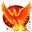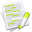#wx.lib.agw.floatspin.FixedPoint¶

FixedPoint objects support decimal arithmetic with a fixed number of digits (called the object’s precision) after the decimal point. The number of digits before the decimal point is variable & unbounded.

The precision is user-settable on a per-object basis when a FixedPoint is constructed, and may vary across FixedPoint objects. The precision may also be changed after construction via FixedPoint.set_precision(p). Note that if the precision of a FixedPoint is reduced via FixedPoint.set_precision(), information may be lost to rounding.

Example:

>>> x = FixedPoint("5.55")  # precision defaults to 2
>>> print(x)
5.55
>>> x.set_precision(1)      # round to one fraction digit
>>> print(x)
5.6
>>> print(FixedPoint("5.55", 1))  # same thing setting to 1 in constructor
5.6
>>> repr(x) #  returns constructor string that reproduces object exactly
"FixedPoint('5.6', 1)"
>>>


When FixedPoint objects of different precision are combined via + - * /, the result is computed to the larger of the inputs’ precisions, which also becomes the precision of the resulting FixedPoint object. Example:

>>> print FixedPoint("3.42") + FixedPoint("100.005", 3)
103.425
>>>


When a FixedPoint is combined with other numeric types (ints, floats, strings representing a number) via + - * /, then similarly the computation is carried out using – and the result inherits – the FixedPoint’s precision. Example:

>>> print(FixedPoint(1) / 7)
0.14
>>> print(FixedPoint(1, 30) / 7)
0.142857142857142857142857142857
>>>


The string produced by str(x) (implictly invoked by print) always contains at least one digit before the decimal point, followed by a decimal point, followed by exactly x.get_precision() digits. If x is negative, str(x) == “-“.

The FixedPoint constructor can be passed an int, long, string, float, FixedPoint, or any object convertible to a float via float() or to an integer via int(). Passing a precision is optional; if specified, the precision must be a non-negative int. There is no inherent limit on the size of the precision, but if very very large you’ll probably run out of memory.

Note that conversion of floats to FixedPoint can be surprising, and should be avoided whenever possible. Conversion from string is exact (up to final rounding to the requested precision), so is greatly preferred. Example:

>>> print(FixedPoint(1.1e30))
1099999999999999993725589651456.00
>>> print(FixedPoint("1.1e30"))
1100000000000000000000000000000.00
>>>


##Class Hierarchy¶Inheritance diagram for class FixedPoint:

##Methods Summary¶

 __init__ Default class constructor. copy Create a copy of the current FixedPoint. frac Returns fractional portion as a FixedPoint. get_precision Return the precision of this FixedPoint. set_precision Change the precision carried by this FixedPoint to precision.

##Class API¶

class FixedPoint(object)

FixedPoint objects support decimal arithmetic with a fixed number of digits (called the object’s precision) after the decimal point. The number of digits before the decimal point is variable & unbounded.

The precision is user-settable on a per-object basis when a FixedPoint is constructed, and may vary across FixedPoint objects. The precision may also be changed after construction via FixedPoint.set_precision(p). Note that if the precision of a FixedPoint is reduced via FixedPoint.set_precision(), information may be lost to rounding.

Example:

>>> x = FixedPoint("5.55")  # precision defaults to 2
>>> print(x)
5.55
>>> x.set_precision(1)      # round to one fraction digit
>>> print(x)
5.6
>>> print(FixedPoint("5.55", 1))  # same thing setting to 1 in constructor
5.6
>>> repr(x) #  returns constructor string that reproduces object exactly
"FixedPoint('5.6', 1)"
>>>


When FixedPoint objects of different precision are combined via + - * /, the result is computed to the larger of the inputs’ precisions, which also becomes the precision of the resulting FixedPoint object. Example:

>>> print FixedPoint("3.42") + FixedPoint("100.005", 3)
103.425
>>>


When a FixedPoint is combined with other numeric types (ints, floats, strings representing a number) via + - * /, then similarly the computation is carried out using – and the result inherits – the FixedPoint’s precision. Example:

>>> print(FixedPoint(1) / 7)
0.14
>>> print(FixedPoint(1, 30) / 7)
0.142857142857142857142857142857
>>>


The string produced by str(x) (implictly invoked by print) always contains at least one digit before the decimal point, followed by a decimal point, followed by exactly x.get_precision() digits. If x is negative, str(x) == “-“.

The FixedPoint constructor can be passed an int, long, string, float, FixedPoint, or any object convertible to a float via float() or to an integer via int(). Passing a precision is optional; if specified, the precision must be a non-negative int. There is no inherent limit on the size of the precision, but if very very large you’ll probably run out of memory.

Note that conversion of floats to FixedPoint can be surprising, and should be avoided whenever possible. Conversion from string is exact (up to final rounding to the requested precision), so is greatly preferred. Example:

>>> print(FixedPoint(1.1e30))
1099999999999999993725589651456.00
>>> print(FixedPoint("1.1e30"))
1100000000000000000000000000000.00
>>>


### Methods¶

__init__(self, value=0, precision=DEFAULT_PRECISION)

Default class constructor.

Parameters
• value – the initial value;

• precision – must be an int >= 0, and defaults to DEFAULT_PRECISION.

copy(self)

Create a copy of the current FixedPoint.

frac(self)

Returns fractional portion as a FixedPoint.

Note

this equality holds true:

x = x.frac() + int(x)


get_precision(self)

Return the precision of this FixedPoint.

Note

The precision is the number of decimal digits carried after the decimal point, and is an int >= 0.

set_precision(self, precision=DEFAULT_PRECISION)

Change the precision carried by this FixedPoint to precision.

Parameters

precision – must be an int >= 0, and defaults to DEFAULT_PRECISION.

Note

If precision is less than this FixedPoint’s current precision, information may be lost to rounding.